ALL

Page: (Previous)   1  2  3  4  (Next)
ALL

### M

#### MAT-HS.F-IF.09

 MAT-HS Targeted Standards(F) Concept: Function(IF) Domain: Interpreting FunctionsCluster: Analyze functions using different representations. MAT-HS.F-IF.09* Compare properties of two functions each represented in a different way (algebraically, graphically, numerically in tables, or by verbal descriptions).

## Student Learning Targets:

• I can
• I can

• I can

### Skills (Performance) Targets

• I can compare the key features of two functions that are represented in different ways.

• I can
• I can

## Proficiency Scale

 Score Description Sample Activity 4.0 (advanced) In addition to achieving level 3.0 content, the student makes in-depth inferences and applications that go beyond what was taught Given the function, y = 3x + 2 create the following: A graph that is increasing at the same rate. A table that is increasing at a lesser rate A verbal description that is increasing at a greater rate. Graph 2x + 3y = 6, 2x + 3y = 12, and 2x + 3y = 18 in the same coordinate plane. . How are the lines from part (a) related? . As C increases, what happens to the graph of 2x + 3y = C 3.5 In addition to Score 3.0 performance, the student demonstrates in-depth inferences and applications regarding the more complex content with partial success. 3.0 (proficient) The student can: compare properties of two functions each represented in a different way: algebraically graphically numerically in tables verbal descriptions The student exhibits no major errors or omissions.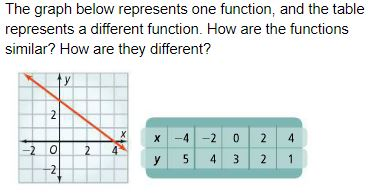The ordered pairs below represent one function, and the verbal description represents a different function. How are the functions similar? How are they different?   (0, 2), (1, 4), (2, 6), (3, 8)   This function has a slope of - ½ and a y-intercept of -32.5 The student demonstrates no major errors or omissions regarding the simpler details and processes (Score 2.0 content) and partial knowledge of the more complex ideas and processes (Score 3.0 content). 2.0 (progressing) There are no major errors or omissions regarding the simpler details and processes as the student: can recognize and recall terminology such as: equation, maximum, minimum, x and y intercept, rate of change, slope can identify properties of a function, but can not compare with another function However, the student exhibits major errors or omissions regarding the more complex ideas and processes.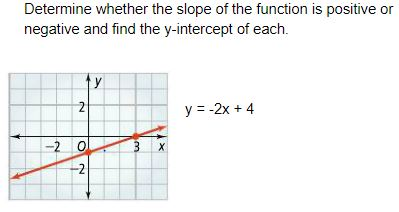1.5 The student demonstrates partial knowledge of the simpler details and processes (Score 2.0 content) but exhibits major errors or omissions regarding the more complex ideas and procedures (Score 3.0 content). 1.0 (beginning) With help, a partial understanding of some of the simpler details and processes and some of the more complex ideas and processes. - 0.5 With help, the student demonstrates a partial understanding of some of the simpler details and processes (Score 2.0 content) but not the more complex ideas and processes (Score 3.0 content).

## Alg II Comparing Functions Proficiency Scale

Score   Description Sample Activity

4.0

In addition to Score 3.0, the student demonstrates in-depth inferences and applications regarding more complex material that go beyond end of instruction expectations.

Which of the functions have a larger increasing interval on the domain from [-2, 3]?

f(x)=ex-3+2   g(x)=x3-4x

3.5 In addition to Score 3.0 performance, the student demonstrates in-depth inferences and applications regarding the more complex content with partial success.

3.0

(proficient)

The student can:
• compare the key features of two functions that are represented in different ways.

The student exhibits no major errors or omissions.

Determine which of the functions presented has the largest maximum value.

f(x)=2x2-28x+90

g(x)=(x+3)(x-2)

Compare the two functions below.  Determine the x-intercept they both share.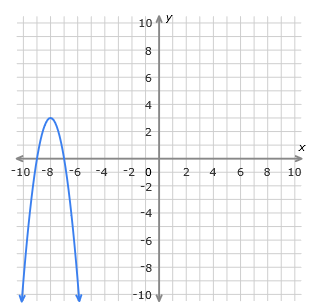x y -14 -2 -7 0 0 2 7 4
2.5 The student demonstrates no major errors or omissions regarding the simpler details and processes (Score 2.0 content) and partial knowledge of the more complex ideas and processes (Score 3.0 content).

2.0

(progressing)

There are no major errors or omissions regarding the simpler details and processes as the student can:
• classify a function given a table, graph, or equation.

• find the key features of a graph, a table, or an equation.
• recognize and recall basic terminology, such as:
• linear
• vertex
• exponential
• intercept
• maximum/minimum
• line of symmetry
• increasing/decreasing intervals
• opens up/opens down

However, the student exhibits major errors or omissions regarding the more complex ideas and processes.

Match the correct equation to the correct graph.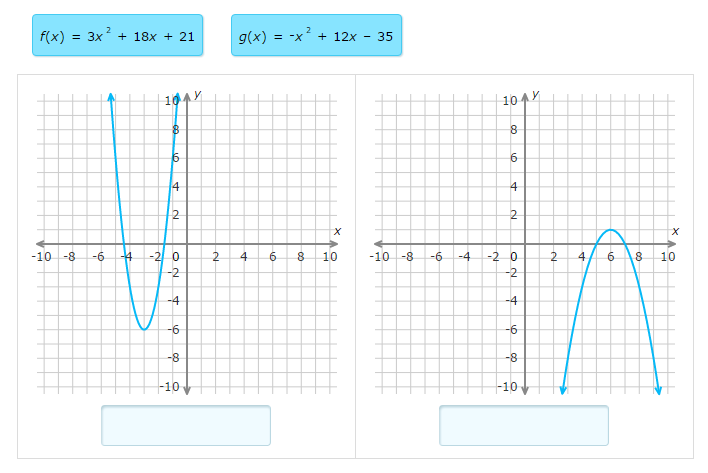Given the two equations, decide which function has the greater slope.     f(x)=2x+9
 x y -2 -3 0 -2 2 -1 4 0
1.5 The student demonstrates partial knowledge of the simpler details and processes (Score 2.0 content) but exhibits major errors or omissions regarding the more complex ideas and procedures (Score 3.0 content).

1.0

(beginning)

With help, the student demonstrates a partial understanding of some of the simpler details and processes (Score 2.0 content) and some of the more complex ideas and processes (Score 3.0 content).
0.5 With help, the student demonstrates a partial understanding of some of the simpler details and processes (Score 2.0 content) but not the more complex ideas and processes (Score 3.0 content).
0.0 Even with help, the student demonstrates no understanding or skill.

## Resources

 Web Vocab

#### MAT-HS.F-LE.01

 MAT-HS Targeted Standards(F) Concept: Function(LE) Domain: Linear, Quadratic and Exponential ModelsCluster: CCC MAT-HS.F-LE.01 Distinguish between situations that can be modeled with linear functions and with exponential functions. a. Prove that linear functions grow by equal differences over equal intervals; exponential functions grow by equal factors over equal intervals. b. Recognize situations in which one quantity changes at a constant rate per unit interval relative to another. c. Recognize situations in which a quantity grows or decays by a constant percent rate per unit interval relative to another.

## Student Learning Targets:

• I can
• I can

### Reasoning Targets

• I can determine a situation as linear or exponential by examining rates of change between data points.

### Skills (Performance) Targets

• I can show there is a constant difference in a linear function over equal intervals.
• I can show there is a constant multiplier in an exponential function over equal intervals.
• I can describe situations where one quantity changes at a constant rate per unit interval relative to another.
• I can describe situations where one quantity grows or decays by a constant multiplier per unit interval relative to another.

• I can
• I can

## Proficiency Scale

 Score Description Sample Activity 4.0 In addition to Score 3.0, the student demonstrates in-depth inferences and applications regarding more complex material that go beyond end of instruction expectations. - 3.5 In addition to Score 3.0 performance, the student demonstrates in-depth inferences and applications regarding the more complex content with partial success. 3.0 “The Standard.” The student demonstrates no major errors or omissions regarding any of the information and processes that were end of instruction expectations. - 2.5 The student demonstrates no major errors or omissions regarding the simpler details and processes (Score 2.0 content) and partial knowledge of the more complex ideas and processes (Score 3.0 content). 2.0 The student demonstrates no major errors or omissions regarding the simpler details and processes but exhibits major errors or omissions regarding the more complex ideas and processes (Score 3.0 content). - 1.5 The student demonstrates partial knowledge of the simpler details and processes (Score 2.0 content) but exhibits major errors or omissions regarding the more complex ideas and procedures (Score 3.0 content). 1.0 With help, the student demonstrates a partial understanding of some of the simpler details and processes (Score 2.0 content) and some of the more complex ideas and processes (Score 3.0 content). - 0.5 With help, the student demonstrates a partial understanding of some of the simpler details and processes (Score 2.0 content) but not the more complex ideas and processes (Score 3.0 content). 0.0 Even with help, the student demonstrates no understanding or skill. -

## Resources

 Web Vocab constant difference constant multiplier

#### MAT-HS.F-LE.02

 MAT-HS Targeted Standards(F) Concept: Function(LE) Domain: Linear, Quadratic and Exponential ModelsCluster: Construct and compare linear and exponential models and solve problems MAT-HS.F-LE.02 Construct linear functions given a graph, table, a description or two input-output pairs given their relationship. **Algebra will focus on point-slope and intercepts as relationships.

## Student Learning Targets:

• I can
• I can

• I can
• I can

### Skills (Performance) Targets

• I can write the equation of a line given a relationship given two characteristics.

• I can
• I can

## Proficiency Scale

 Score Description Sample Activity 4.0 (advanced) In addition to Score 3.0, the student demonstrates in-depth inferences and applications regarding more complex material that go beyond end of instruction expectations. Suppose a 5-minute overseas call costs $5.91 and a 10-minute call costs$10.86.  The cost of the call and the length of the call are related.  The cost of each minute is constant.         A.  What is the cost, c, of a call of m minutes duration?        B.  How long can you talk on the phone if you have \$12 to spend? 3.5 In addition to Score 3.0 performance, the student demonstrates in-depth inferences and applications regarding the more complex content with partial success. 3.0 (proficient) The student can: Write linear functions given... point/slope 2 points parallel/perpendicular lines and a slope Write an equation in point-slope form for the line that has the given slope and that passes through the given point. m = 3  (0, -2) m = -2  (5,1) Write an equation in slope-intercept form that passes through the given points. (2,3) & (1,5) (5, -2) & (-16, 4) Write an equation in slope-intercept form for the line that passes through the given point and is PARALLEL to the given line. (4,5)  y = -2x + 4 (-7,3)  x = 4 Write an equation in slope-intercept form for the line that passes through the given point and is PERPENDICULAR to the given line. (5, -1)  y = 4x - 7 (4, -2)  y = 3 Write the equation of the function represented by the graph in slope-intercept form.2.5 The student demonstrates no major errors or omissions regarding the simpler details and processes (Score 2.0 content) and partial knowledge of the more complex ideas and processes (Score 3.0 content). 2.0 (progressing) The student demonstrates no major errors or omissions regarding the simpler details and processes but exhibits major errors or omissions regarding the more complex ideas and processes (Score 3.0 content).   Can find slope between 2 points Write the equation of a line given the slope and y-intercept Find parallel/perpendicular slopes from an equation   However, the student exhibits major errors or omissions regarding the more complex ideas and processes. Write the equation of the line given a slope of 4 and a y-intercept of -5. Find the slope of the line that goes through  (-2, 5) and (3, 8). What is the slope of the line parallel to y=3x + 8 What is the slope of the line perpendicular to y=-4x - 6 1.5 The student demonstrates partial knowledge of the simpler details and processes (Score 2.0 content) but exhibits major errors or omissions regarding the more complex ideas and procedures (Score 3.0 content). 1.0 (beginning) With help, the student demonstrates a partial understanding of some of the simpler details and processes (Score 2.0 content) and some of the more complex ideas and processes (Score 3.0 content). - 0.5 With help, the student demonstrates a partial understanding of some of the simpler details and processes (Score 2.0 content) but not the more complex ideas and processes (Score 3.0 content). 0.0 Even with help, the student demonstrates no understanding or skill. -

## Resources

 Web Vocab

#### MAT-HS.F-LE.03

 MAT-HS Targeted Standards(F) Concept: Function(LE) Domain: Linear, Quadratic and Exponential ModelsCluster: Construct and compare linear and exponential models and solve problems MAT-HS.F-LE.03 Observe using graphs and tables that a quantity increasing exponentially eventually exceeds a quantity increasing linearly, quadratically, or (more generally) as a polynomial function.

## Student Learning Targets:

• I can
• I can

• I can
• I can

### Skills (Performance) Targets

• I can use graphs and tables to make the connection that a quantity increasing exponentially eventually exceeds a quantity increasing linearly, quadratically, or any other polynomial function.

• I can
• I can

## Proficiency Scale

 Score Description Sample Activity 4.0 In addition to Score 3.0, the student demonstrates in-depth inferences and applications regarding more complex material that go beyond end of instruction expectations. - 3.5 In addition to Score 3.0 performance, the student demonstrates in-depth inferences and applications regarding the more complex content with partial success. 3.0 “The Standard.” The student demonstrates no major errors or omissions regarding any of the information and processes that were end of instruction expectations. - 2.5 The student demonstrates no major errors or omissions regarding the simpler details and processes (Score 2.0 content) and partial knowledge of the more complex ideas and processes (Score 3.0 content). 2.0 The student demonstrates no major errors or omissions regarding the simpler details and processes but exhibits major errors or omissions regarding the more complex ideas and processes (Score 3.0 content). - 1.5 The student demonstrates partial knowledge of the simpler details and processes (Score 2.0 content) but exhibits major errors or omissions regarding the more complex ideas and procedures (Score 3.0 content). 1.0 With help, the student demonstrates a partial understanding of some of the simpler details and processes (Score 2.0 content) and some of the more complex ideas and processes (Score 3.0 content). - 0.5 With help, the student demonstrates a partial understanding of some of the simpler details and processes (Score 2.0 content) but not the more complex ideas and processes (Score 3.0 content). 0.0 Even with help, the student demonstrates no understanding or skill. -

## Resources

 Web Vocab

#### MAT-HS.F-LE.04

 MAT-HS Targeted Standards(F) Concept: Function(LE) Domain: Linear, Quadratic and Exponential ModelsCluster: Construct and compare linear and exponential models and solve problems MAT-HS.F-LE.04 Use logarithms to express the solution to abct=d where a, c, and d are real numbers and b is a positive real number. Evaluate the logarithm using technology when appropriate.

## Student Learning Targets:

### Knowledge Targets

• I can define a logarithm.
• I can recall properties of logarithms and exponents.
• I can recognize common logarithms and natural logarithms.
• I can identify an asymptote.
• I can recall the change of base formula.

### Reasoning Targets

• I can convert from a logarithm to exponential expressions.
• I can identify an asymptote.
• I can expand and condense logarithms.
• I can evaluate logarithms and exponential expressions.
• I can distinguish between a natural log and common log.

### Skills (Performance) Targets

• I can apply basic properties of exponents and logarithms to rewrite algebraic expressions.
• I can graph logarithmic and exponential functions.
• I can solve logarithmic and exponential equations.
• I can graph and determine the equation of an asymptote.
• I can express the solution to an exponential function as a logarithm.
• I can evaluate logarithms using technology.

• I can
• I can

## Alg II Solve Exponential Equations Proficiency Scale

Score   Description Sample Activity

4.0

In addition to Score 3.0, the student demonstrates in-depth inferences and applications regarding more complex material that go beyond end of instruction expectations.

Describe the error in the following work: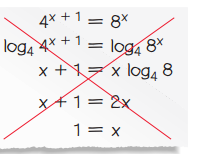3.5 In addition to Score 3.0 performance, the student demonstrates in-depth inferences and applications regarding the more complex content with partial success.

3.0

(proficient)

The student can:

• solve an exponential equation using logarithms.

The student exhibits no major errors or omissions.

 Solve the following equation.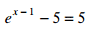2.5 The student demonstrates no major errors or omissions regarding the simpler details and processes (Score 2.0 content) and partial knowledge of the more complex ideas and processes (Score 3.0 content).

2.0

(progressing)

There are no major errors or omissions regarding the simpler details and processes as the student can:
• recognize and recall specific terminology such as :
• exponent
• logarithm
• Euler number (e)
• base
• identify an exponential equation.
• use inverse operations.
• evaluate a logarithmic expression.
However, the student exhibits major errors or omissions regarding the more complex ideas and processes.

Which of the following is an exponential equation?

1.    43x-2=1

2.    2x+1=8

3.   3x2-2=45
1.5 The student demonstrates partial knowledge of the simpler details and processes (Score 2.0 content) but exhibits major errors or omissions regarding the more complex ideas and procedures (Score 3.0 content).

1.0

(beginning)

With help, the student demonstrates a partial understanding of some of the simpler details and processes (Score 2.0 content) and some of the more complex ideas and processes (Score 3.0 content).
0.5 With help, the student demonstrates a partial understanding of some of the simpler details and processes (Score 2.0 content) but not the more complex ideas and processes (Score 3.0 content).
0.0 Even with help, the student demonstrates no understanding or skill.

## Resources

 Web Vocab

#### MAT-HS.F-LE.05

 MAT-HS Targeted Standards(F) Concept: Function(LE) Domain: Linear, Quadratic and Exponential ModelsCluster: Interpret expressions for functions in terms of the situation they model. MAT-HS.F-LE.05 Interpret the parameters in a linear, quadratic, or exponential function in terms of a context.

## Student Learning Targets:

• I can
• I can

• I can
• I can

### Skills (Performance) Targets

• I can explain the meaning of the coefficients, constants, factors, exponents, and intercepts in a linear, quadratic or exponential function in terms of a context.

• I can
• I can

## Proficiency Scale

 Score Description Sample Activity 4.0 In addition to Score 3.0, the student demonstrates in-depth inferences and applications regarding more complex material that go beyond end of instruction expectations. - 3.5 In addition to Score 3.0 performance, the student demonstrates in-depth inferences and applications regarding the more complex content with partial success. 3.0 “The Standard.” The student demonstrates no major errors or omissions regarding any of the information and processes that were end of instruction expectations. - 2.5 The student demonstrates no major errors or omissions regarding the simpler details and processes (Score 2.0 content) and partial knowledge of the more complex ideas and processes (Score 3.0 content). 2.0 The student demonstrates no major errors or omissions regarding the simpler details and processes but exhibits major errors or omissions regarding the more complex ideas and processes (Score 3.0 content). - 1.5 The student demonstrates partial knowledge of the simpler details and processes (Score 2.0 content) but exhibits major errors or omissions regarding the more complex ideas and procedures (Score 3.0 content). 1.0 With help, the student demonstrates a partial understanding of some of the simpler details and processes (Score 2.0 content) and some of the more complex ideas and processes (Score 3.0 content). - 0.5 With help, the student demonstrates a partial understanding of some of the simpler details and processes (Score 2.0 content) but not the more complex ideas and processes (Score 3.0 content). 0.0 Even with help, the student demonstrates no understanding or skill. -

## Resources

 Web Vocab coefficient constant parameter

#### MAT-HS.F-TF.01

 MAT-HS Targeted Standards(F) Concept: Function(TF) Domain: Trigonometric FunctionsCluster: Extend the domain of trigonometric functions using the unit circle. MAT-HS.F-TF.01 Understand that the radian measure of an angle is the length of the arc on the unit circle subtended by the angle.

## Student Learning Targets:

### Knowledge Targets

• I can identify when the length of an arc subtended by an angle is the same length as the radius of the circle then the angle is 1 radian.

• I can
• I can

### Skills (Performance) Targets

• I can explain that the length of the arc of a subtended angle on the unit circle is the radian measure of the angle.

• I can
• I can

## Proficiency Scale

 Score Description Sample Activity 4.0 In addition to Score 3.0, the student demonstrates in-depth inferences and applications regarding more complex material that go beyond end of instruction expectations. - 3.5 In addition to Score 3.0 performance, the student demonstrates in-depth inferences and applications regarding the more complex content with partial success. 3.0 “The Standard.” The student demonstrates no major errors or omissions regarding any of the information and processes that were end of instruction expectations. - 2.5 The student demonstrates no major errors or omissions regarding the simpler details and processes (Score 2.0 content) and partial knowledge of the more complex ideas and processes (Score 3.0 content). 2.0 The student demonstrates no major errors or omissions regarding the simpler details and processes but exhibits major errors or omissions regarding the more complex ideas and processes (Score 3.0 content). - 1.5 The student demonstrates partial knowledge of the simpler details and processes (Score 2.0 content) but exhibits major errors or omissions regarding the more complex ideas and procedures (Score 3.0 content). 1.0 With help, the student demonstrates a partial understanding of some of the simpler details and processes (Score 2.0 content) and some of the more complex ideas and processes (Score 3.0 content). - 0.5 With help, the student demonstrates a partial understanding of some of the simpler details and processes (Score 2.0 content) but not the more complex ideas and processes (Score 3.0 content). 0.0 Even with help, the student demonstrates no understanding or skill. -

## Resources

 Web Vocab

#### MAT-HS.F-TF.02

 MAT-HS Targeted Standards(F) Concept: Function(TF) Domain: Trigonometric FunctionsCluster: Extend the domain of trigonometric functions using the unit circle. MAT-HS.F-TF.02 Explain how the unit circle in the coordinate plane enables the extension of trigonometric functions to all real numbers, interpreted as radian measures of angles traversed counterclockwise around the unit circle.

## Student Learning Targets:

• I can
• I can

• I can
• I can

### Skills (Performance) Targets

• I can use the unit circle to extend the domain of the trigonometric functions beyond quadrant I and interpret the positive angles as counterclockwise rotations in radian form and negative angles as clockwise rotations in radian form.
• I can explain the connection between the corresponding trigonometric function values in quadrant I and all trigonometric function values resulting from any radian angle measures.

• I can
• I can

## Proficiency Scale

 Score Description Sample Activity 4.0 In addition to Score 3.0, the student demonstrates in-depth inferences and applications regarding more complex material that go beyond end of instruction expectations. - 3.5 In addition to Score 3.0 performance, the student demonstrates in-depth inferences and applications regarding the more complex content with partial success. 3.0 “The Standard.” The student demonstrates no major errors or omissions regarding any of the information and processes that were end of instruction expectations. - 2.5 The student demonstrates no major errors or omissions regarding the simpler details and processes (Score 2.0 content) and partial knowledge of the more complex ideas and processes (Score 3.0 content). 2.0 The student demonstrates no major errors or omissions regarding the simpler details and processes but exhibits major errors or omissions regarding the more complex ideas and processes (Score 3.0 content). - 1.5 The student demonstrates partial knowledge of the simpler details and processes (Score 2.0 content) but exhibits major errors or omissions regarding the more complex ideas and procedures (Score 3.0 content). 1.0 With help, the student demonstrates a partial understanding of some of the simpler details and processes (Score 2.0 content) and some of the more complex ideas and processes (Score 3.0 content). - 0.5 With help, the student demonstrates a partial understanding of some of the simpler details and processes (Score 2.0 content) but not the more complex ideas and processes (Score 3.0 content). 0.0 Even with help, the student demonstrates no understanding or skill. -

## Resources

 Web Vocab

#### MAT-HS.F-TF.03

 MAT-HS Targeted Standards(F) Concept: Function(TF) Domain: Trigonometric FunctionsCluster: Extend the domain of trigonometric functions using the unit circle. MAT-HS.F-TF.03 Use special triangles to det ermine geometrically the values of sine, cosine, tangent for π /3, π /4 and π /6, and use the unit circle to expr ess the values of sine, cosine, and tangent for π-x, π+x, and 2 π-x in terms of their values for x, where x is any real number.

• I can
• I can

• I can
• I can

• I can
• I can

• I can
• I can

## Proficiency Scale

 Score Description Sample Activity 4.0 In addition to Score 3.0, the student demonstrates in-depth inferences and applications regarding more complex material that go beyond end of instruction expectations. - 3.5 In addition to Score 3.0 performance, the student demonstrates in-depth inferences and applications regarding the more complex content with partial success. 3.0 “The Standard.” The student demonstrates no major errors or omissions regarding any of the information and processes that were end of instruction expectations. - 2.5 The student demonstrates no major errors or omissions regarding the simpler details and processes (Score 2.0 content) and partial knowledge of the more complex ideas and processes (Score 3.0 content). 2.0 The student demonstrates no major errors or omissions regarding the simpler details and processes but exhibits major errors or omissions regarding the more complex ideas and processes (Score 3.0 content). - 1.5 The student demonstrates partial knowledge of the simpler details and processes (Score 2.0 content) but exhibits major errors or omissions regarding the more complex ideas and procedures (Score 3.0 content). 1.0 With help, the student demonstrates a partial understanding of some of the simpler details and processes (Score 2.0 content) and some of the more complex ideas and processes (Score 3.0 content). - 0.5 With help, the student demonstrates a partial understanding of some of the simpler details and processes (Score 2.0 content) but not the more complex ideas and processes (Score 3.0 content). 0.0 Even with help, the student demonstrates no understanding or skill. -

## Resources

 Web Vocab

#### MAT-HS.F-TF.04

 MAT-HS Targeted Standards(F) Concept: Function(TF) Domain: Trigonometric FunctionsCluster: Extend the domain of trigonometric functions using the unit circle. MAT-HS.F-TF.04 Use the unit circle to explain symmetry (odd and even) and periodicity of trigonometric functions.

• I can
• I can

• I can
• I can

• I can
• I can

• I can
• I can

## Proficiency Scale

 Score Description Sample Activity 4.0 In addition to Score 3.0, the student demonstrates in-depth inferences and applications regarding more complex material that go beyond end of instruction expectations. - 3.5 In addition to Score 3.0 performance, the student demonstrates in-depth inferences and applications regarding the more complex content with partial success. 3.0 “The Standard.” The student demonstrates no major errors or omissions regarding any of the information and processes that were end of instruction expectations. - 2.5 The student demonstrates no major errors or omissions regarding the simpler details and processes (Score 2.0 content) and partial knowledge of the more complex ideas and processes (Score 3.0 content). 2.0 The student demonstrates no major errors or omissions regarding the simpler details and processes but exhibits major errors or omissions regarding the more complex ideas and processes (Score 3.0 content). - 1.5 The student demonstrates partial knowledge of the simpler details and processes (Score 2.0 content) but exhibits major errors or omissions regarding the more complex ideas and procedures (Score 3.0 content). 1.0 With help, the student demonstrates a partial understanding of some of the simpler details and processes (Score 2.0 content) and some of the more complex ideas and processes (Score 3.0 content). - 0.5 With help, the student demonstrates a partial understanding of some of the simpler details and processes (Score 2.0 content) but not the more complex ideas and processes (Score 3.0 content). 0.0 Even with help, the student demonstrates no understanding or skill. -

## Resources

 Web Vocab

Page: (Previous)   1  2  3  4  (Next)
ALL